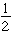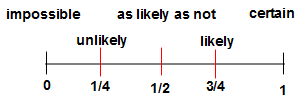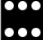# Probability

Probability measures the likelihood of an event occurring. This measure is based on the total number of outcomes possible and can be expressed as a fraction or a decimal from 0-1, or a percent from 0-100%.

As you can see by the number line below, if the probability is close to 0, the event is unlikely. If the probability is close to 1, the event is very likely. When the probability is nearor 0.5, then that even is as likely as not. Probability of an event is written: P (event).### Example

Chelle is going to select a random card from 12 cards. They are numbered 1 to 12. What is the probability that she will choose a card with a number less than 4? Determine if this event is likely or unlikely.

• Count the number of favorable outcomes.
• Numbers less than 4: 1, 2, 3
• Count the number of total outcomes.
• 12
• Find the probability P(number less than 4).
• 3/12 or 1/4
• Determine if this event is likely or unlikely.
• The probability of choosing a card with a number less than 4 is unlikely (less than one half).

### Example

A bag contains 7 red marbles, 3 purple marbles, and 2 blue marbles. What is the probability of picking a blue marble?

• Count the number of favorable outcomes
• 2 blue marbles
• Count the total number of outcomes
• 12 marbles
• Find the probability P(blue marble)
• 2/12 or 1/6
• The probability of P(blue marble) is 1/6 or unlikely.

Let’s try a few more:Determine whether each of the following events is impossible, unlikely, as likely as not, likely, or certain. Check your answers.

1. Roll an odd number on a fair number cube (1-6)
2. Roll a number less than 3 on a fair number cube (1-6)
3. Roll a number equal to or greater than 3 on a fair number cube (1-6)
4. Roll a number less than 7 on a fair number cube (1-6)
5. Roll a number greater than 6 on a fair number cube (1-6)

## Complementary Events

Now, what about those outcomes that are not the expected or favorable event. These events are called complementary events. In other words, the sum of the event and its complement will always equal 1.

## P(event) + P(complement) = 1

1. Roll an odd number on a fair number cube (1-6)
P(event) = P(odd) = 3/6 = 1/2
P(compliment) = P(not odd) = 3/6 = 1/2
1/2 + 1/2 = 1
2. Roll a number less than 3 on a fair number cube (1-6)
3. Roll a number equal to or greater than 3 on a fair number cube (1-6)
4. Roll a number less than 7 on a fair number cube (1-6)
5. Roll a number greater than 6 on a fair number cube (1-6)

## PROBABILITY WORD PROBLEMS

1. Bailey’s math teacher almost always introduces a new topic by showing a short video clip. Bailey’s class just completed a topic on Friday. Should she expect the teacher to show a video clip next week?

SolutionSince they finished studying a topic, they will be learning about a new topic. Since the  teacher almost always starts off with a video clip, it is likely that the teacher will show a video clip next week.

2. Mike’s music club meets on Wednesday afternoons. How likely is it that Mike is at the movies on Wednesday afternoon?

SolutionSince the music club meets on Wednesdays, it is unlikely that Mike is at the movies on Wednesday afternoon.

3.Teri rides the bus to school if she gets up by 7:30 A.M. Teri gets up at 7:30 A.M. about half of the time so can you estimate the probability that Teri will ride the bus to school?

SolutionThe probability that she will ride the bus is ½, so it is just as likely as not that she will ride the bus to school.

Watch the video to see more examples of probability.

## Probability Practice

#### Determine whether each event is impossible, unlikely, as likely as not, likely, or certain.

1. A spinner with six equal sections labeled 1 through 6 landing on a number less than seven.
2. Flipping a coin and having it land with the tails side facing up.
3. Picking a purple ball from a bag containing 7 purple balls and 1 green ball.
4. Rolling a number greater than 7 on a number cube with sides numbered one through six.
5. Picking the 8 of hearts from a standard deck of playing cards.
6. A spinner with 8 equal sections labeled 1 through 8 landing on a number less than nine.
7. Flipping a coin and having it land with the heads side facing up
8. Picking a red marble from a bag containing 4 red marbles and 4 white marbles.
9. Rolling a sum of 14 with two number cubes with sides one through six.